# RD Sharma Class 7 ex 2.1 Solutions Chapter 2 Fractions

In this chapter, we provide RD Sharma Class 7 ex 2.1 Solutions Chapter 2 Fractions for English medium students, Which will very helpful for every student in their exams. Students can download the latest RD Sharma Class 7 ex 2.1 Solutions Chapter 2 Fractions Maths pdf, Now you will get step by step solution to each question.

## RD Sharma Solutions for Class 7 Chapter 2 Fractions Ex 2.1 Download PDF

### Question: 1

Compare the following fractions by using the symbol > or < or =;

### Solution:

(i) We have,

Taking the LCM of 9 and 13, we get,

9 x 13 = 117

Now, we convert the given fractions to equivalent fractions by making the denominators 117,

As we know, 91 > 72

Hence, 79 > 813

(ii)  We have,

The given fractions are equivalent fractions as the denominators are equal,

And we know that, 11 > 5

Therefore, 119 > 59

(iii)  We have,

Taking the LCM of 41 and 30, we get,

41 x 30 = 1230

Now, we convert the given fractions to equivalent fractions by making the denominators 1230,

Now, we clearly know 1110 > 779

Hence, 3741 > 1930

(iv) We have,

Taking the LCM of 15 and 105, we get,

5 x 3 x 7 = 105

Now, we convert the given fractions to equivalent fractions by making the denominators 105,

### Question: 2

Arrange the following fractions in ascending order:

### Solution:

(i)  We have,

Taking the LCM of 8, 6, 8, 4 and 3, we get,

2 x 4 x 3 = 24

Now, we convert the given fractions to equivalent fractions by making the denominators 24,

We know that, 8 < 9 < 12 < 18 < 20

Hence, 13 < 38 < 24 < 68 < 56

(ii) We have,

Taking the LCM of 6, 8, 12 and 16, we get,

2 x 2 x 2 x 2 x 3 = 48

Now, we convert the given fractions to equivalent fractions by making the denominators 48,

We know that, 12 < 15 < 18 < 32

### Question: 3

Arrange the following fractions in descending order:

### Solution:

(i)  We have,

Taking the LCM of 5, 10, 15 and 20, we get,

5 x 2 x 2 x 3 = 60

Now, we convert the given fractions to equivalent fractions by making the denominators 48

As we know 51 > 48 > 44 > 42

(ii) We have,

Taking the LCM of 7, 35, 14 and 28, we get,

7 x 5 x 2 x 2 = 140

Now, we convert the given fractions to equivalent fractions by making the denominators 140

As we know 40 > 44 > 65 > 90

### Question: 4

Write the equivalent fractions of 3/5

### Solution:

Multiplying or dividing both the numerator and denominator by the same number,

so that the fraction keeps its value.

So the equivalent fractions of 3/5 are

are the five equivalent fractions of 3/5

Find the sum:

### Solution:

(i)  We have,

Taking the LCM of 8 and 10, we get,

2 x 4 x 5 = 40

Now, we convert the given fractions to equivalent fractions by making the denominators 40

(ii) We have,

Taking out the LCM of 4 and 5, we get,

4 x 5 = 20

Now, we convert the given fractions to equivalent fractions by making the denominators 20

(iii)  We have,

Taking out the LCM of 6 and 4, we get,

2 x 2 x 3 = 12

Now, we convert the given fractions to equivalent fractions by making the denominators 12

(iv) We have,

Taking out the LCM of 5, 10 and 15, we get,

5 x 2 x 3 = 30

Now, we convert the given fractions to equivalent fractions by making the denominators 30

### Question: 6

Find the difference of

### Solution:

(i)  We have,

Taking out the LCM of 24 and 16, we get,

2 x 2 x 2 x 2 x 3 = 48

Now, we convert the given fractions to equivalent fractions by making the denominators 48

(ii)  We have, 6 and 23/3

The difference between 6 and 23/3

(iii)  We have,

Taking out the LCM of 25 and 20, we get,

5 x 5 x 4 = 100

Now, we convert the given fractions to equivalent fractions by making the denominators 100

The difference between both the fractions are

(iv) We have,

Taking out the LCM of 10 and 15, we get,

2 x 3 x 5 = 30

Now, we convert the given fractions to equivalent fractions by making the denominators 30

The difference between both the fractions are

### Question: 7

Find the difference:

### Solution:

(i)  We have,

Taking out the LCM of 7 and 11, we get,

7 x 11 = 77

Now, we convert the given fractions to equivalent fractions by making the denominators 77

The difference between both the fractions are

(ii)  We have,

(iii) We have,

(iv) We have,

Taking out the LCM of 10 and 15, we get,

2 x 3 x 5 = 30

Now, we convert the given fractions to equivalent fractions by making the denominators 30

Simplify:

### Solution:

(i) We have,

Taking out the LCM of 3, 6 and 9, we get,

3 x 3 x 2 = 18

Now, we convert the given fractions to equivalent fractions by making the denominators 18, we get,

(ii)  We have,

(iii) We have,

Taking out the LCM of 6, 8 and 12, we get,

2 x 2 x 2 x 3 = 48

Now, we convert the given fractions to equivalent fractions by making the denominators 48, we get,

### Question: 9

What should be added toto get 12?

### Solution:

We have,

Let x be the number added to 38/7 to get 12

Therefore,

x + 387 = 12

### Question: 10

What should be added to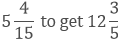?

### Solution:

Let x be the number added to 79/15 to get 63/5

Taking out the LCM of 5 and 15, we get,

3 x 5 = 15

Now, we convert the given fractions to equivalent fractions by making the denominators 15, we get,

### Question: 11

Suman studies for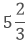hours daily. She devotes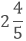hours of her time for science and mathematics. How much time does she devote for other subjects?

### Solution:

Given,

Suman studies forhours daily

She devoteshours of her time for science and mathematics. Let x be time she devotes for other subjects.

Taking out the LCM of 3 and 5, we get,

3 x 5 = 15

Now, we convert the given fractions to equivalent fractions by making the denominators 48, we get,

### Question: 12

A piece of wire of length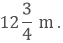If it is cut into two pieces in such a way that the length of one piece is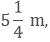what is the length of the other piece?

### Solution:

A piece of wire of lengthone piece isLet the length of other piece be x m.

### Question: 13

A rectangular piece of paper is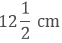long andwide. Find its perimeter?

### Solution:

Given,

A rectangular piece of paper islong andwide

Perimeter = 2(length + width)

### Question: 14

In a “magic square”, the sum of numbers in each row, in each column and along the diagonal is same. Is this a “magic square”?

### Solution:

Given,

Therefore, the sum of numbers in each row, in each column and along the diagonal is same and the sum is 15/11.

### Question: 15

The cost of Mathematics book is Rs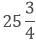and that of science book isWhich costs more and by how much?

### Solution:

Given,

The cost of Mathematics book is Rsand that of science book isWe need to compare the cost of mathematics and science book,

Taking out the LCM of 4 and 2, we get,

2 x 2 = 4

Now, we convert the given fractions to equivalent fractions by making the denominators 4, we get

= 103/4

As we know, 103 > 82

Hence, the cost of mathematics book is more than that of the cost of the science book.

### Question: 16

Provide the number in the box [] and also give its simplest form in each of the following:

### Solution:

All Chapter RD Sharma Solutions For Class 7 Maths

I think you got complete solutions for this chapter. If You have any queries regarding this chapter, please comment on the below section our subject teacher will answer you. We tried our best to give complete solutions so you got good marks in your exam.